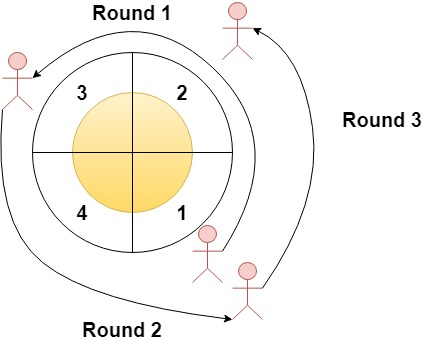# 圆形赛道上经过次数最多的扇区

## 1560. 圆形赛道上经过次数最多的扇区 (Easy)```输入：n = 4, rounds = [1,3,1,2]

1 --> 2 --> 3（阶段 1 结束）--> 4 --> 1（阶段 2 结束）--> 2（阶段 3 结束，即本场马拉松结束）

```输入：n = 2, rounds = [2,1,2,1,2,1,2,1,2]

```

```输入：n = 7, rounds = [1,3,5,7]

```

• `2 <= n <= 100`
• `1 <= m <= 100`
• `rounds.length == m + 1`
• `1 <= rounds[i] <= n`
• `rounds[i] != rounds[i + 1]` ，其中 `0 <= i < m`

[数组]